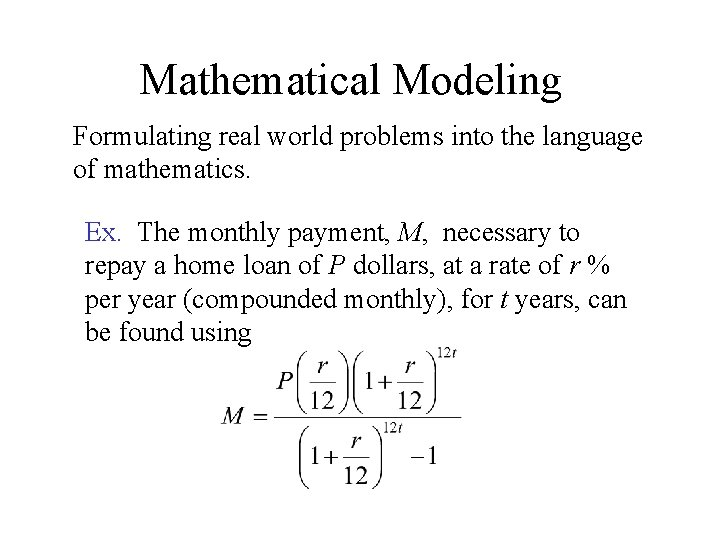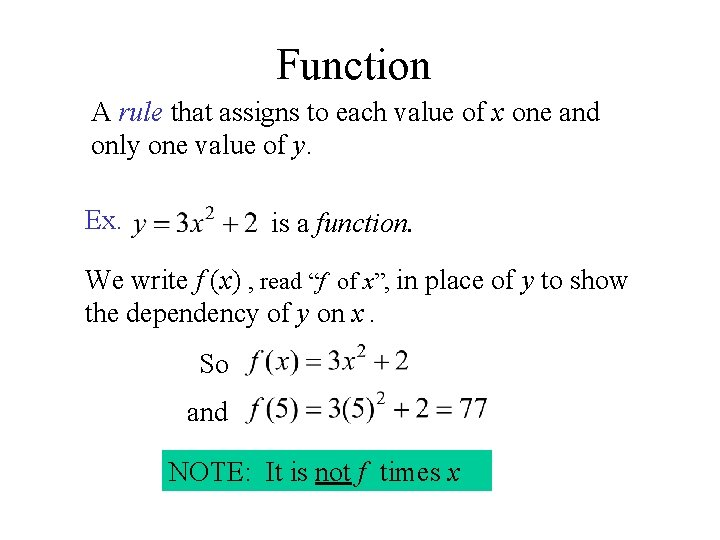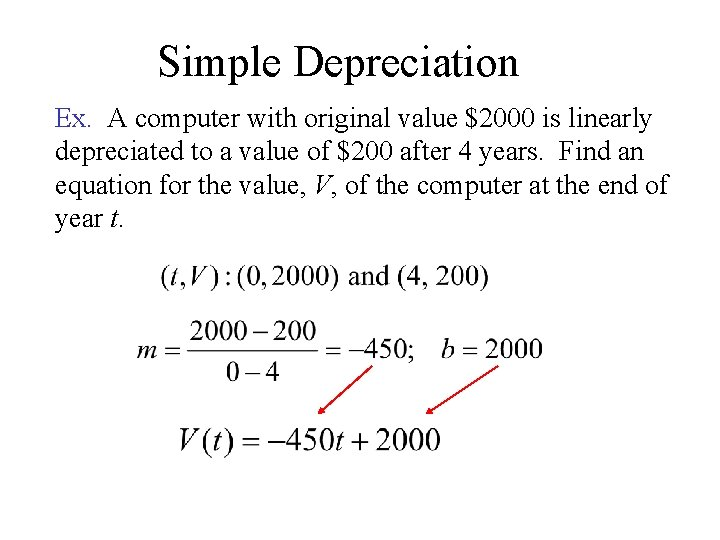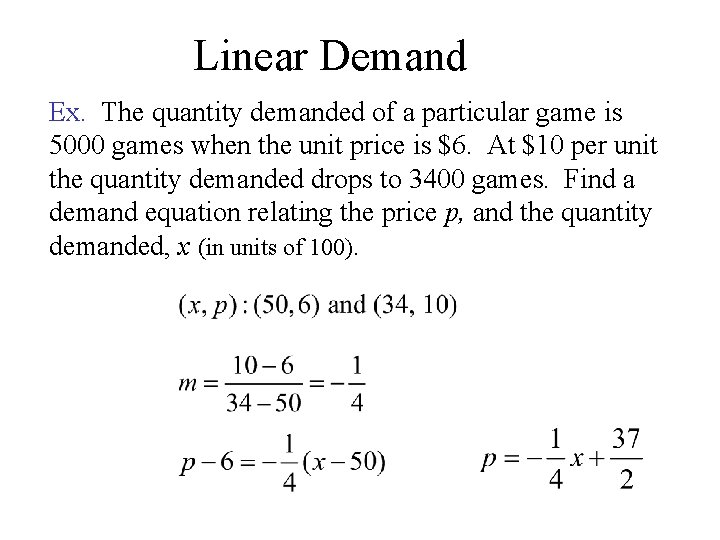# Section 1 3 Linear Functions and Mathematical Modeling

• Slides: 7Section 1. 3 Linear Functions and Mathematical ModelingMathematical Modeling Formulating real world problems into the language of mathematics. Ex. The monthly payment, M, necessary to repay a home loan of P dollars, at a rate of r % per year (compounded monthly), for t years, can be found usingFunction A rule that assigns to each value of x one and only one value of y. Ex. is a function. We write f (x) , read “f of x”, in place of y to show the dependency of y on x. So and NOTE: It is not f times xLinear Function A linear function can be expressed in the form m and b are constants Can be used for • Simple Depreciation • Linear Supply and Demand Functions • Linear Cost, Revenue, and Profit FunctionsSimple Depreciation Ex. A computer with original value \$2000 is linearly depreciated to a value of \$200 after 4 years. Find an equation for the value, V, of the computer at the end of year t.Cost, Revenue, and Profit Functions Ex. A shirt producer has a fixed monthly cost of \$3600. If each shirt has a cost of \$3 and sells for \$12 find: a. The cost function Cost: C(x) = 3 x + 3600 where x is the number of shirts produced. b. The revenue function Revenue: R(x) = 12 x where x is the number of shirts sold. c. The profit from 900 shirts Profit: P(x) = Revenue – Cost = 12 x – (3 x + 3600) = 9 x – 3600 P(900) = 9(900) – 3600 = \$4500Linear Demand Ex. The quantity demanded of a particular game is 5000 games when the unit price is \$6. At \$10 per unit the quantity demanded drops to 3400 games. Find a demand equation relating the price p, and the quantity demanded, x (in units of 100).